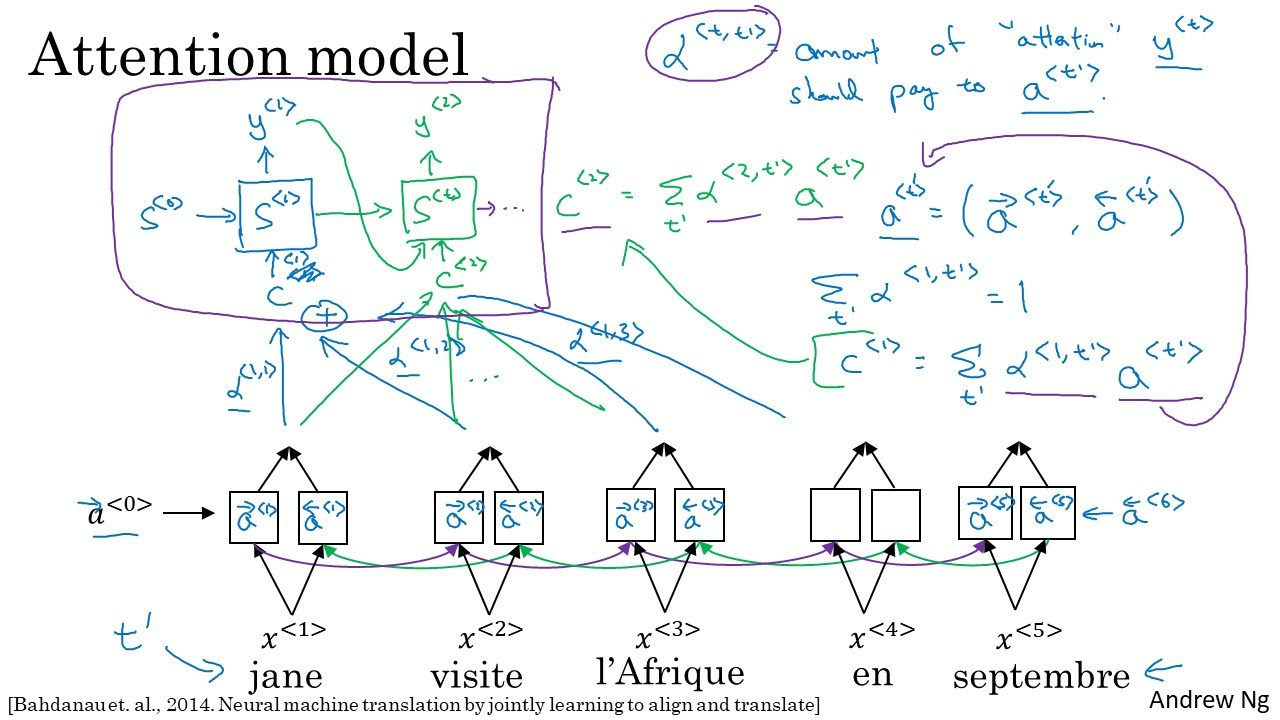# Attention Model

Categories:

Updated:

https://arxiv.org/abs/1409.0473

In sequence to sequence model, we first encoded the input sequence and decoded it. For machine translation task, it is like memorizing the whole sentence before translating it. But actually it is more natural to read some part of the sentence, translate it, read next part, translate, and so on.

Attention model was invented with this intuition.We put ‘decoding’ RNN on top of the ‘encoding’ RNN. Activation $s^{<t>}$ in the decoding RNN is inputted with $x^{<t>}$ and $c^{<t>}$.

This $c^{<t>}$ is computed with attention weight $\alpha^{<t,t’>}$ . Attention weight $\alpha^{<t,t’>}$ is the amount of attention $\hat{y}^{<t>}$ should pay to $a^{<t>}$ .

I’ll give precise formulas of attention model works.

### 2. With activation from the encoding BRNN and previous state of decoding RNN, compute $e$

$W_e$ and $b_e$ are a trainable parameter so will be optimized by gradient descent.

### 5. Compute normal RNN layer

where $\hat{y}_{class}^{<t-1>}$ is a predicted class one-hot representation of $\hat{y}^{<t-1>}$

Categories: